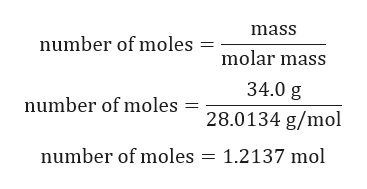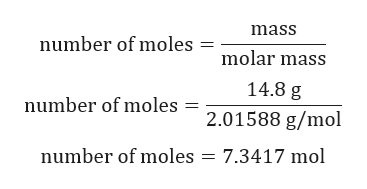# Be sure to answer all parts. Ammonia is produced by the reaction of nitrogen and hydrogen according to the equation N2(g) + 3H2(g) → 2NH3(g) Calculate the mass of ammonia produced when 34.0 g of nitrogen react with 14.8 g of hydrogen.   g NH3 Which is the excess reactant and how much of it will be left over when the reaction is complete?  hydrogen  nitrogen  g

Question
Be sure to answer all parts.

Ammonia is produced by the reaction of nitrogen and hydrogen according to the equation

N2(g) + 3H2(g) → 2NH3(g)

Calculate the mass of ammonia produced when 34.0 g of nitrogen react with 14.8 g of hydrogen.

g NH3

Which is the excess reactant and how much of it will be left over when the reaction is complete?
 hydrogen nitrogen

g
check_circleExpert Solution
Step 1

Given:

Mass of N2 = 34.0 g

Mass of H2 = 14.8 g

N2(g) + 3H2(g) → 2NH3(g)

Step 2

Calculation for number of moles of N2:help_outlineImage Transcriptionclosemass number of moles molar mass 34.0 g number of moles = 28.0134 g/mol number of moles = 1.2137 mol fullscreen
Step 3

Calculation for number of mo...help_outlineImage Transcriptionclosemass number of moles molar mass 14.8 g number of moles 2.01588 g/mol number of moles 7.3417 mol fullscreen

### Want to see the full answer?

See Solution

#### Want to see this answer and more?

Solutions are written by subject experts who are available 24/7. Questions are typically answered within 1 hour*

See Solution
*Response times may vary by subject and question
Tagged in

### General Chemistry